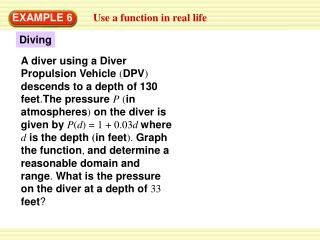DownloadDownload PresentationDiving

Diving

Télécharger la présentationDiving

- - - - - - - - - - - - - - - - - - - - - - - - - - - E N D - - - - - - - - - - - - - - - - - - - - - - - - - - -
Presentation Transcript

1. EXAMPLE 6 Use a function in real life Diving A diver using a Diver Propulsion Vehicle (DPV) descends to a depth of 130 feet.The pressure P(in atmospheres) on the diver is given by P(d) = 1 + 0.03d where dis the depth (in feet). Graph the function, and determine a reasonable domain and range. What is the pressure on the diver at a depth of 33 feet?

2. Use the vertical line test EXAMPLE 6 SOLUTION The graph ofP(d) is shown.Because the depth varies from0feet to130feet, areasonable domain is0≤d≤130. The minimum value of P(d)isP(0) = 1, and the maximum value of P(d)isP(130) = 4.9. So,a reasonablerange is 1 ≤P(d) ≤4.9.

3. ANSWER At a depth of 33 feet, the pressure on the diver is P(33) = 1 + 0.03(33) 2 atmospheres, which you can verify from the graph. Use the vertical line test EXAMPLE 6

4. SOLUTION Because the depth varies from0feet to35,800feet, areasonable domain is0 ≤d≤35,800. The minimum value of P(d)isP(0) = 1, and the maximum value of P(d)isP(35,800) = 1075.So,a reasonablerange is 1≤P(d) ≤1075. for Example 6 GUIDED PRACTICE 7. In 1960, the deep-sea vessel Trieste descended to an estimated depth of 35,800 feet. Determine a reasonable domain and range of the function P(d) in Example 6 for this trip.